Next: SPECTRAL FACTORIZATION Up: HILBERT TRANSFORM Previous: Instantaneous envelope

## Instantaneous frequency

The phase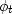of a complex-valued signal gt = ut+ivt is defined by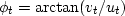.The instantaneous frequency is.Before forming the derivative, recall the definition of a complex logarithm of g: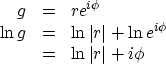(12)
Hence,.The instantaneous frequency is(13)
For a signal that is a pure sinusoid, such as,equation (13) clearly gives the right answer. When various frequencies are simultaneously present, we can hope that (13) gives a sensible average.

Trouble can arise in (13) when the denominator g gets small, which happens whenever the envelope of the signal gets small. This difficulty can be overcome by careful smoothing. Rationalize the denominator by multiplying by the conjugate signal, and then smooth locally a little (as indicated by the summation sign below):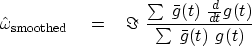(14)
(Those of you who have studied quantum mechanics may recognize the notion of expectation of an operator.'' You will also see why the wave probability function of quantum physics must be complex valued: as a consequence of the analytic signal eliminating negative frequencies from the average. If the negative frequencies were not eliminated, then the average frequency would be zero.)

What range of times should be smoothed in equation (14)? Besides the nature of the data, the appropriate smoothing depends on the method of representing.To prepare a figure, I implementedby multiplying by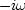.(This is more accurate than finite differences at high frequencies, but has the disadvantage that the discontinuity in slope at the Nyquist frequency gives an extended transient in the time domain.) The result is shown in Figure 5.node
Figure 5
A sum of three sinusoids (top), unsmoothed instantaneous frequency (middle), and smoothed instantaneous frequency (bottom).

Inspection of the figure shows that smoothing is even more necessary for instantaneous frequency than for envelopes, and this is not surprising because the presence ofmakes the signal rougher. Particularly notice times in the range 400-512 where the sinusoids are truncated. There the unsmoothed instantaneous frequency becomes a large rapid oscillation near the Nyquist frequency. This roughness is nicely controlled by (1,2,1) smoothing.

It is gratifying to see that a spike added to the sinusoids (at point 243) causes a burst of high frequency. Also interesting to notice is where an oscillation approaches the axis and then turns away just before or just after crossing the axis.

An example of instantaneous frequency applied to field data is shown in Figure 6.frequency
Figure 6
A field profile (left), instantaneous frequency smoothed only with (1,2,1) (middle), and smoothed more heavily (right).

The instantaneous-frequency idea can also be applied to the space axis. This will be more easily understood by readers familiar with the methodology of imaging and migration. Instead of temporal frequency,we compute the spatial frequency.Figure 7 gives an example. Analogously, we could make plots of local dip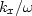.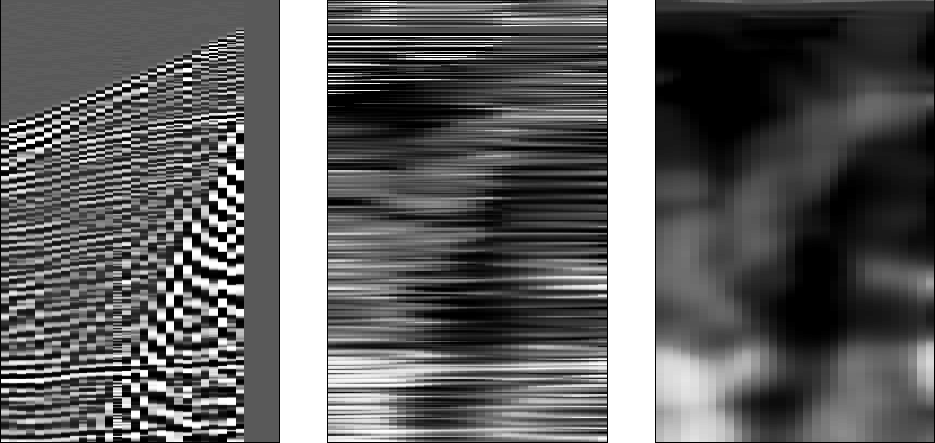kx
Figure 7
A field profile (left), kx smoothed over x only (center), and smoothed over t and x (right).

## EXERCISES:

1. Let ct be a causal complex-valued signal. How does X(Z) change in equation (2), and how must Y(Z) in equation (3) be deduced from X(Z)?
2. Figure 3 shows a Hilbert-transform pair, the real and imaginary parts of the Fourier transform of a causal response. Describe the causal response.
3. Given Y(Z)=Q(Z)X(Z), prove that the envelope of yt is the same as the envelope of xt.
4. Using partial fractions, convolve the waveform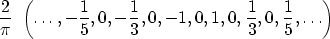with itself. What is the interpretation of the fact that the result is? (HINT:.)
5. Using the fast-Fourier-transform matrix, we can represent the quadrature filterby the column vector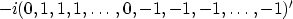Multiply this vector into the inverse-transform matrix to show that the transform is proportional to.What is the scale factor? Sketch the scale factor for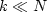, indicating the limit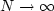.(HINT: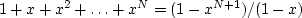.)Next: SPECTRAL FACTORIZATION Up: HILBERT TRANSFORM Previous: Instantaneous envelope
Stanford Exploration Project
10/21/1998